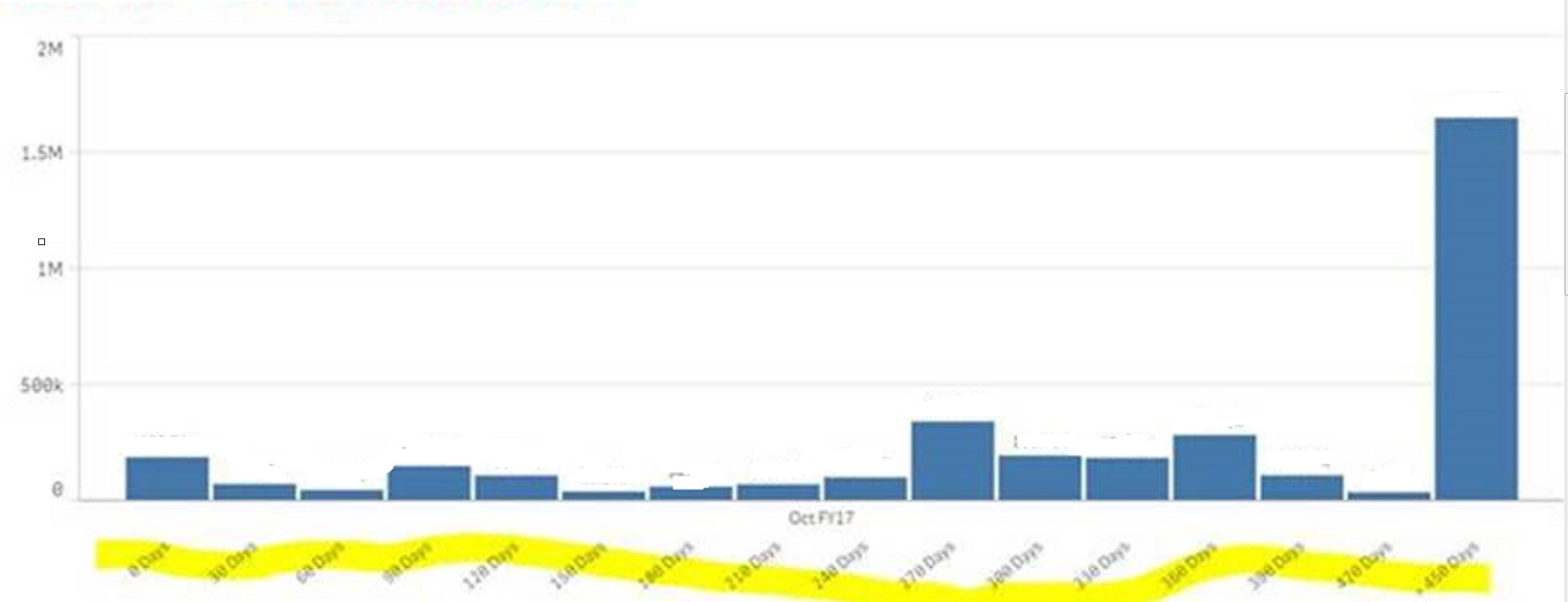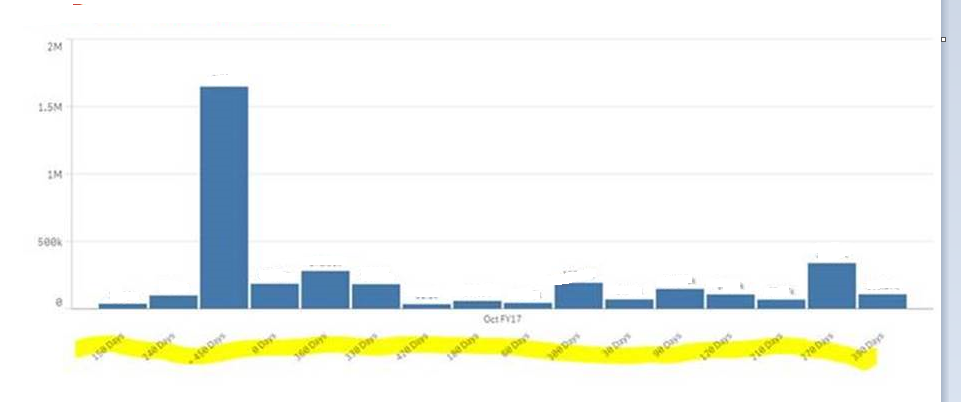# New to Qlik Sense

Discussion board where members can get started with Qlik Sense.

Announcements
Qlik® Product Spotlight: Discover what’s possible. Get more from our products.
See for yourself. Register today.Not applicable

## How can we fix the sorting of the visualization?

Sorting works fine when I select a particular month as a filter  but does not work when no filter is selected. How can we fix the sorting issue in visualization?

With Filter selected:With No filter Selected:11 RepliesMVP

## Re: How can we fix the sorting of the visualization?

Would you be able to share some information on how are you sorting this? Is it using an expression? Can you share your expression?Not applicable

## Re: How can we fix the sorting of the visualization?

Sorting includes three dimensions FY Month(Auto Sorting), Timing(Expression=Month_end_metric_date_key) and cases(Auto)

and Timing data contains following expression

if(report_metric_key = 3,

if(((num#(mid(month_end_metric_date_key,5,2)) - num#(mid(month_end_date_key,5,2))) * 30) + ((num#(mid(month_end_metric_date_key,1,4)) - num#(mid(month_end_date_key,1,4))) * 360) = 450,
'> 450 Days',
((num#(mid(month_end_metric_date_key,5,2)) - num#(mid(month_end_date_key,5,2))) * 30) + ((num#(mid(month_end_metric_date_key,1,4)) - num#(mid(month_end_date_key,1,4))) * 360) & ' Days'
)
)

Honored Contributor III

## Re: How can we fix the sorting of the visualization?

Can you share sample app?Not applicable

## Re: How can we fix the sorting of the visualization?

Sorry, working on server ...no sample data

New Contributor III

## Re: How can we fix the sorting of the visualization?

Try this:

in sorting Tab

deselect the auto selection and mark sort by expression

in expression ='num(ExampleDate)'

Regards,

Dixit panchal.MVP

## Re: How can we fix the sorting of the visualization?

Try this as your calculated dimension:

If(report_metric_key = 3,

If(((Num#(Mid(month_end_metric_date_key,5,2)) - Num#(Mid(month_end_date_key,5,2))) * 30) + ((Num#(Mid(month_end_metric_date_key,1,4)) - Num#(Mid(month_end_date_key,1,4))) * 360) = 450,
Dual('> 450 Days', 450),
Dual(((num#(mid(month_end_metric_date_key,5,2)) - num#(mid(month_end_date_key,5,2))) * 30) + ((num#(mid(month_end_metric_date_key,1,4)) - num#(mid(month_end_date_key,1,4))) * 360) & ' Days',

((num#(mid(month_end_metric_date_key,5,2)) - num#(mid(month_end_date_key,5,2))) * 30) + ((num#(mid(month_end_metric_date_key,1,4)) - num#(mid(month_end_date_key,1,4))) * 360))
)
)Not applicable

## Re: How can we fix the sorting of the visualization?

Thank You Sunny . It works absolutely fine. Really Appreciated. and If you could help me with the hardcode visualization Question that I have uploaded?Not applicable

## Re: How can we fix the sorting of the visualization?

Hi Sunny , this code only works when no filter selection is made for year and Month ...I have two linked Visualization which returns no value if I use this code.MVP

## Re: How can we fix the sorting of the visualization?

Was this working before you made the changes I proposed?

Community Browser